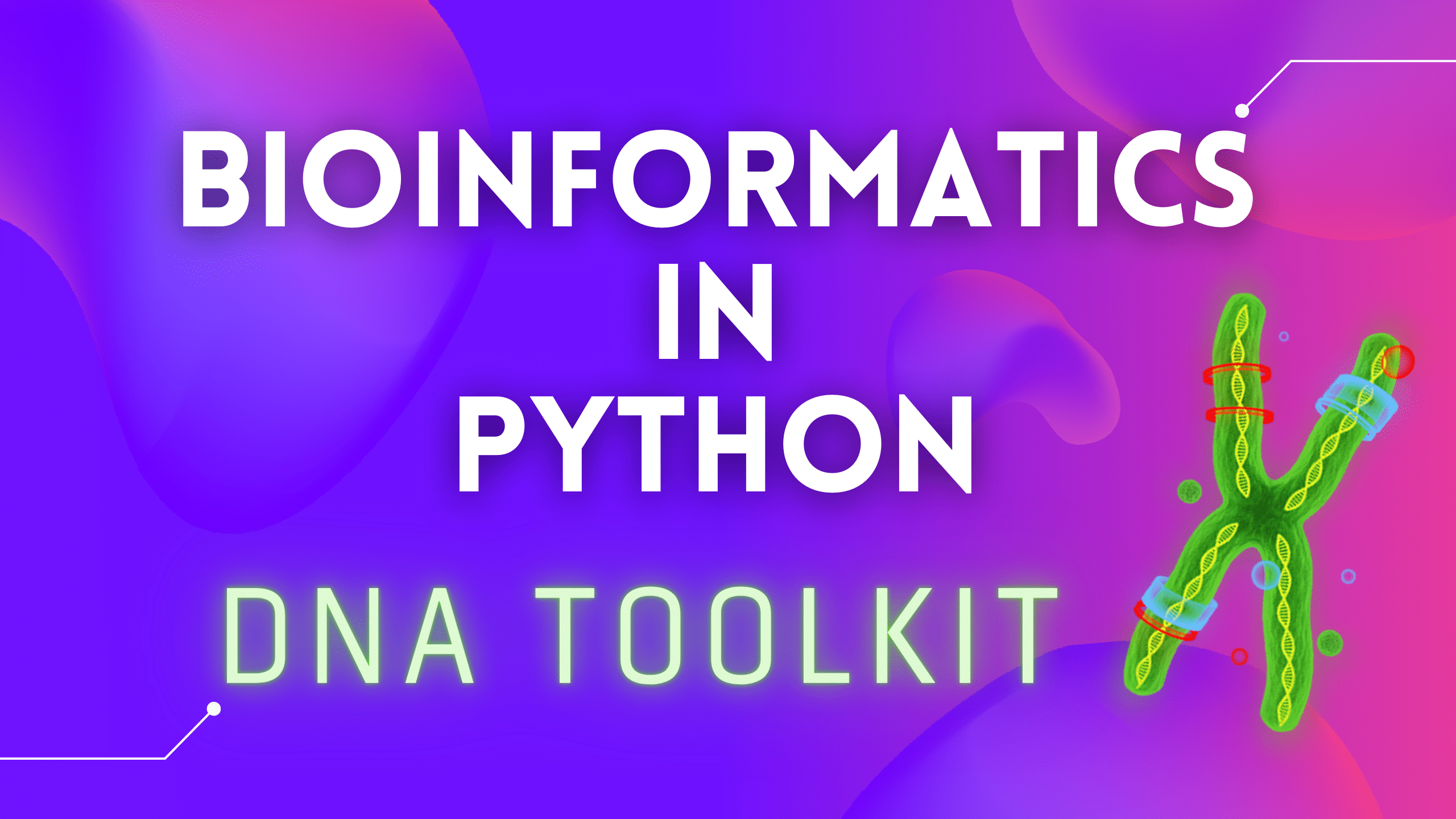# DNA Toolkit Part 3: GC Content CalculationIn this article, we are going to implement two functions. The first function will count G and C nucleotides (GC Content) in a string, and the second function will use the first function but allow us to specify a ‘window’ size to calculate GC content in.

Here is a Wikipedia excerpt on GC Content:

In molecular biology and geneticsGC-content (or guanine-cytosine content) is the percentage of nitrogenous bases in a DNA or RNA molecule that are either guanine (G) or cytosine (C). This measure indicates the proportion of G and C bases out of an implied four total bases, also including adenine and thymine in DNA and adenine and uracil in RNA.

GC-content may be given for a certain fragment of DNA or RNA or for an entire genome. When it refers to a fragment, it may denote the GC-content of an individual gene or section of a gene (domain), a group of genes or gene clusters, a non-coding region, or a synthetic oligonucleotide such as a primer.

So open our `dna_toolkit.py` file and add the first function:

```def gc_content(seq):
"""GC Content in a DNA/RNA sequence"""
return round((seq.count('C') + seq.count('G')) / len(seq) * 100)```

This is a very simple function as we only use built-in Python functionality. We search for character ‘C’ and ‘G’ using `count()` method of the sequence we pass to this function (seq). Then we use the most basic calculation to get the percentage (%). Basically: the amount of G and C, divided by the length of the whole sequence (seq) and then multiplied by a 100 to get %.

So if we pass “ATCG” to our function, we should get 50% back, if we pass “ATCA”, we get 25% back and we get 75% if we pass “AGCG” for example.

Now, what if we want to count GC content in sub-areas of a DNA sequence? Let’s say we are interested in ‘window’ size 3:

```window = 3
seq = "CGATGAATCTATA"```

So we would have something like that:

`[CGA]TGAATCTATA = 67%CGA[TGA]ATCTATA = 33%CGATGAA[TCT]ATA = 33%CGATGAATCTA[TAA] = 0%`

We can ‘prepare’ our DNA string before we pass it to `gc_content()`, and pass each part of size ‘windows’, but this will take a lot more code and users of our `gc_content()` function will not be happy about having to prepare data before using our function. So let’s fix that and program our second function:

```def gc_content_subsec(seq, k=5):
"""
GC Content in a DNA/RNA sub-sequence length k.
k=20 by default
"""
res = []
for i in range(0, len(seq) - k + 1, k):
subseq = seq[i:i + k]
res.append(gc_content(subseq))
return res```

We are using our original `gc_content()` (line 9) function and we are adding a little bit of logic on top of that to handle the ‘window’, which we call ‘k‘ and we set it to 5 by default, just in case if you forget to pass k to our function.

Our `for` loop makes sure we scan the whole sequence and make k (5 by default) jumps, to replicate the example above. We use string slicing to grab only the part of the string we need, which, again, is of size k (our window). And we accumulator results in a list `res`.

So now if we run this on another test DNA string: “ATATGATAGATAGCCCAGTCCGT“, and a window size 5, we should have this output:

`[20, 20, 60, 60]`

Alright. Now we have our two new functions working, let’s add a proper output to our `main.py` file and run the whole thing:

```print(f' + GC Content: {gc_content(DNAStr)}%\n')
print(f' + GC Content in Subsection k=5: {gc_content_subsec(DNAStr, k=5)}\n')```

If we run our `main.py` file now, we should see something like this:

```Sequence: CTGTCCTTCTCATCTACGGTGATTCCAATGTGGTACATTGCGCTTTTTCA

 + Sequence Length: 50

 + Nucleotide Frequency: {'A': 8, 'C': 13, 'G': 9, 'T': 20}

 + DNA/RNA Transcription: CUGUCCUUCUCAUCUACGGUGAUUCCAAUGUGGUACAUUGCGCUUUUUCA

 + DNA String + Complement + Reverse Complement:
5' CTGTCCTTCTCATCTACGGTGATTCCAATGTGGTACATTGCGCTTTTTCA 3'
||||||||||||||||||||||||||||||||||||||||||||||||||
3' GACAGGAAGAGTAGATGCCACTAAGGTTACACCATGTAACGCGAAAAAGT 5' [Complement]
5' TGAAAAAGCGCAATGTACCACATTGGAATCACCGTAGATGAGAAGGACAG 3' [Rev. Complement]

 + GC Content: 44%

 + GC Content in Subsection k=5: [60, 40, 40, 60, 40, 40, 40, 40, 60, 20]
```